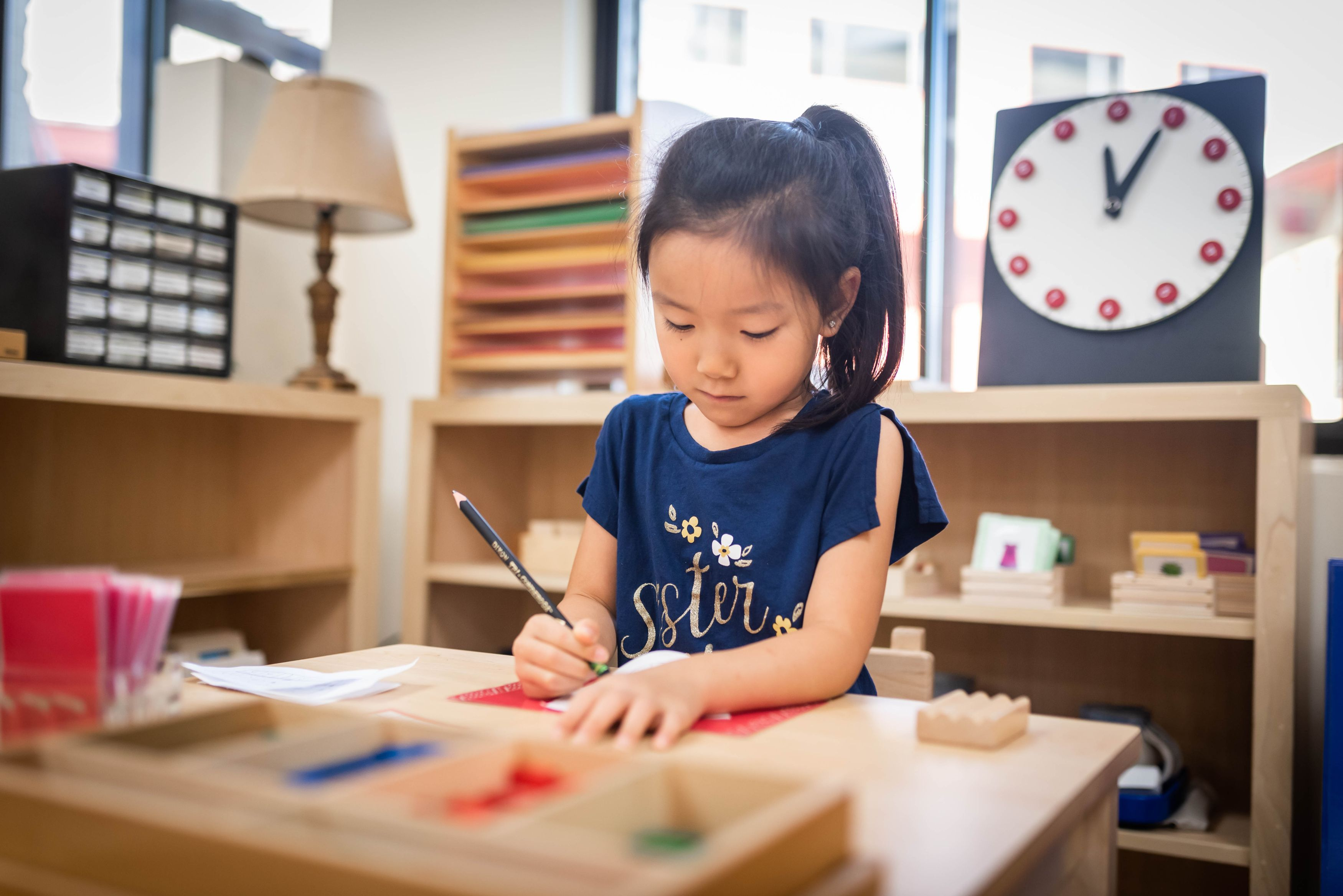Education for life

# Mathematics

The Primary math curriculum utilizes concrete materials to teach the basics of mathematics conceptually, without reliance on rote memorization. Children progress from recognizing and sequencing single digit numbers to the arithmetical operations using up to four-digit numbers.

The math curriculum begins with materials that help children associate the name and symbol for the numerals 0 through 9. Students learn numbers in isolation, the concept of quantity, and corresponding symbols. Students progress to the Spindle Boxes where they learn each number represents a quantity, as well as the concept of absence of quantity, or zero. Cards and Counters gives more practice with quantity (or set formation) while also introducing the idea of odd and even.

Special emphasis is placed on increments of 10, as they are the basis of the decimal system. Older children work with the Golden Beads which concretely represent the units of 1, 10, 100, and 1,000 that make up the decimal system. They practice counting up to 1,000 using the Bead Cabinet material, progressing to skip counting as preparation for multiplication. They also complete a series of lessons to learn addition, subtraction, multiplication, and division. Students are introduced to the beginnings of algebra through the Binomial and Trinomial Cubes, as well as the Decanomial Square.